Functions - AP Calculus: Homeschool Curriculum Chapter Exam

Exam Instructions:

Choose your answers to the questions and click 'Next' to see the next set of questions. You can skip questions if you would like and come back to them later with the yellow "Go To First Skipped Question" button. When you have completed the practice exam, a green submit button will appear. Click it to see your results. Good luck!

Answered 0 of 30

Page 1

Question 4 4. The revenue of a company is represented by R(x) and their costs are represented by C(x). What is the profit function R(x) - C(x)?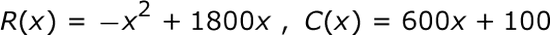Question 5 5. Evaluate f(f(x)). [Yes, it says f(f(x)]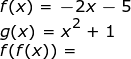Page 2

Question 9 9. In the figure shown below, the graph of the yellow function is a translation of the graph of the blue function. If the expression for the blue function is y(x) = b(x), which of the following is the equation of the yellow function?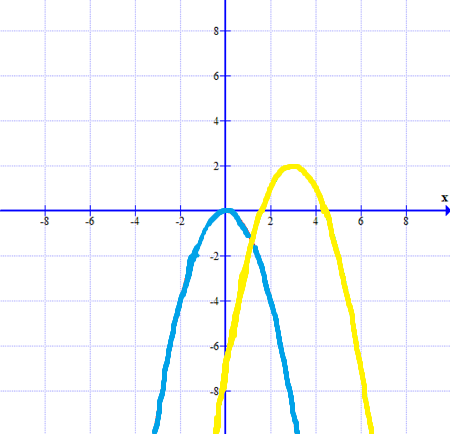Page 4

Question 16 16. What is the range of the function represented by the graph below?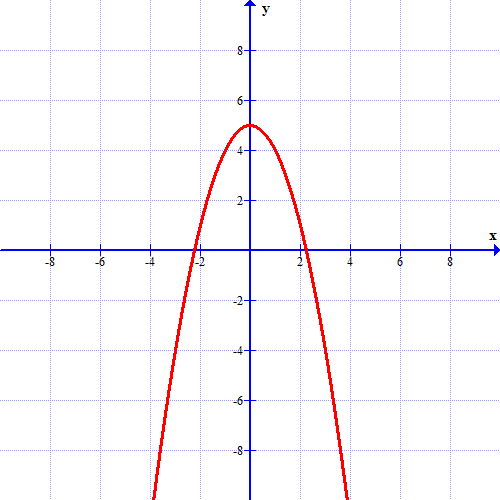Question 17 17. What is the domain of the function below?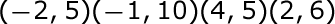Question 19 19. If the graph of f(x) is shown below, which choice represents the graph of f(x + 1) + 2?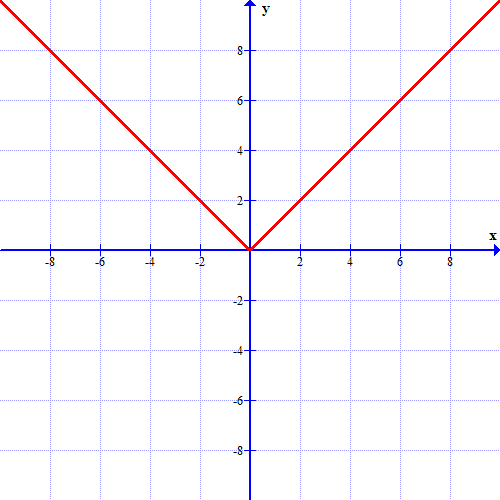Page 5

Question 21 21. Use the graph to find f(f(2)).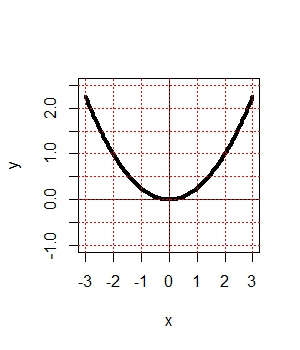Question 25 25. Which kind of transformation would change the purple function below into the yellow one?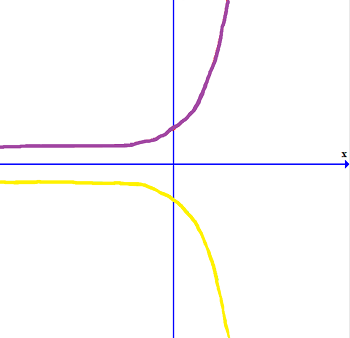Page 6

Question 26 26. Using the function below, evaluate g(7).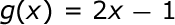Question 28 28. Find the domain of f(x)/g(x), if: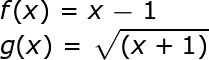Question 29 29. Evaluate.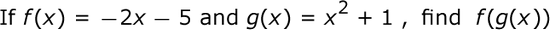Functions - AP Calculus: Homeschool Curriculum Chapter Exam Instructions

Choose your answers to the questions and click 'Next' to see the next set of questions. You can skip questions if you would like and come back to them later with the yellow "Go To First Skipped Question" button. When you have completed the practice exam, a green submit button will appear. Click it to see your results. Good luck!

Support